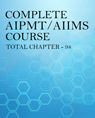×#### Thank you for registering.

One of our academic counsellors will contact you within 1 working day.

Click to Chat

1800-1023-196

+91-120-4616500

CART 0

• 0

MY CART (5)

Use Coupon: CART20 and get 20% off on all online Study Material

ITEM
DETAILS
MRP
DISCOUNT
FINAL PRICE
Total Price: Rs.

There are no items in this cart.
Continue Shopping
```
where to use S proportional to Tsquare and Gallilios odd number ratio in 1D

```
2 years ago

```							we can use the formula s=ut+1/2at^2, when initial velocity of the body is zero and it is moving with an uniform acceleration......................we can use the odd number rule of galileo when a freely falling body is covering equal distances in equal time intervals Let's consider a freely falling body and time intervals of 1 sec. The distance traveled in first interval i.e 1 sec = 1/2 (10) (12) = 5 m The distance traveled in second interval i.e. between 2nd second, = distance traveled in 2 seconds - distance traveled in 1st second = 1/2 (10)(4) - 5 = 15 m Hence we can calculate distance traveled in successive intervals. Now let's try considering ratio of distances in successive intervals, i.e. 15/5 = 3 Similarly if we proceed and find ratio of distance traveled in 3rd second and 2nd second, we'll get 5. This is summarized by Galileo's law of odd numbers, The distances traveled during equal intervals of time, by a body freely falling from rest, stand to one another in the same ratio as the odd numbers beginning with unity.
```
2 years ago
Think You Can Provide A Better Answer ?

## Other Related Questions on AIPMT

View all Questions »### Course Features

• 731 Video Lectures
• Revision Notes
• Previous Year Papers
• Mind Map
• Study Planner
• NCERT Solutions
• Discussion Forum
• Test paper with Video Solution### Course Features

• 728 Video Lectures
• Revision Notes
• Test paper with Video Solution
• Mind Map
• Study Planner
• NCERT Solutions
• Discussion Forum
• Previous Year Exam Questions